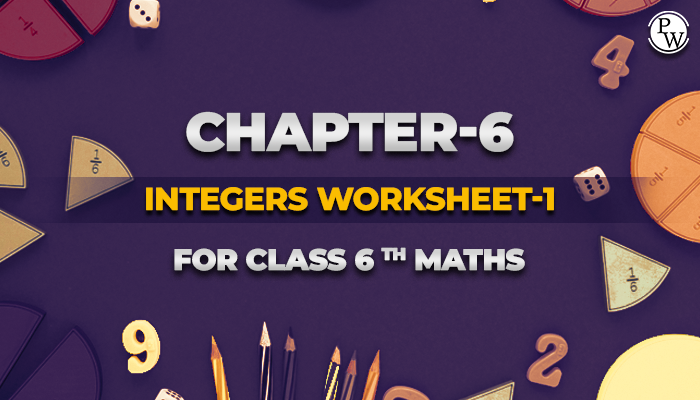# Chapter-6 Integers Worksheet-1 for class 6 maths

#### Worksheets for Class 6 Maths

The academic team of Physics Wallah prepared worksheets and questions for chapter Integers for all students who are in class 6. Physics Wallah questions for class 6 maths chapter Integers consist of carefully selected numerical for class 6 maths and consist of step by step solutions of all questions with answers .

Download worksheet-1 of CBSE class 6 maths chapter Integers and start solving the questions for reference use the solutions. Before solving the worksheet of Physics Wallah it is highly recommended reading the theory of chapter and solve the questions given in your textbook . For additional information related to the subject you can check the Maths Formula section.

Students can also access the Class 6 notes from here and If any students need to take the online test to check their concepts or undertstanding then they can visit Quiz for Integers.## Solve Examples

Q1. The predecessor and successor of -123 are respectively.

1. -124,-125
2. -124,-122
3. -124,-123
4. -125,-126

Ans. Correct answer is 2

The predecessor of -123 = -123 - 1 = -124 and the successor of -123 = -123 + 1 =- 122

Q2.If we add -13 to the difference of -7 and 5. Then we have

1. -24
2. -23
3. -26
4. -25

Ans. Correct answer is 4

Firstly we shall find the difference between -7 and 5. The difference between -7 and 5 = -7 -5 = -12

The required answer = (-13) + (-12)=-25.

Q3. -5 X -4=…….

1. -20
2. 20
3. Both
4. None of them

Ans. Correct answer is 2

(negative integer) x (negative integer)= positive integer.

So,-5 x -4=20

Q4. Simplification of 18 + [3+{7–8+(6-5)+3}-9] is

1. 15
2. -15
3. 14
4. 13

Ans. Correct answer is 1

By the rule of BODMAS,

= 18+[3+{7-8+(6-5)+3}-9]

= 18+[3+{7-8+1+3}-9]

= 18+[3+{7+1+3-8}-9]

= 18+[3+{11-8}-9]

= 18+[3+3-9]

= 18+[6-9]

= 18-3

=15

Q5. If Δ is an operation such that for integers a and b, a Δ b=-a+b+(-2); then 2 Δ -4 equals to

1. -8
2. -7
3. -6
4. 8

Ans. Correct answer is 1

-a Δ b=-a+b+(-2)

Here, a = 2 and b = -4

-2 Δ -4=-2 + (-4) + (-2)

By,the sum of negative integers always negative.

Hence, -2 Δ -4 = -8.

Q6. The sum of two integers is 23. If one integer is -11, the other integer is

1. -34
2. 34
3. 33
4. 35

Ans. Correct answer is 2

Let required integer be x. Then according to question x+(-11)=23

x = 23 + 11 = 34.

Q7. While playing cards, Ram won Rs 79 in the first game, lost Rs 60 in the second game, again won rs 45 in the third game and finally lost Rs 100 in the fourth game. Find his final loss or gain.

1. 36 loss
2. 60 gain
3. 50 loss
4. 70 gain

Ans. Correct answer is 1

Here we shall show negative sign for loss and positive sign for won. So, Ram got=79-60+45-100

= 79 + 45 -60 - 100

= 124 - 160 = -36

negative sign show that Ram got loss of Rs 36.

Q8. The cube of a negative integer is a

1. Positive
2. Negative
3. Nutral
4. None of them

Ans. Correct answer is 2

If the power of any negative integer is odd, then we have always negative answer.

Q9. The following integers in the decreasing order; -4, 7, 0, -2, 3, -6

1. 7, 3, 0,-2,-4, -6
2. -6, -4, -2, 0, 3, 7
3. 7, 3, 0, -1, -2, -4
4. -6, -4, -2, 0, 1, 2

Ans. Correct answer is 1

According to number line, its clear

Q10. The value of 12 / (-3) is

1. -4
2. -3
3. 4
4. 3

Ans. Correct answer is 1

a/b= negative integer, if b is any positive integer. Here b is negative, and then we have -4.

Below link consist of class 6 maths chapter-6 Integers download your free pdf copy from the below